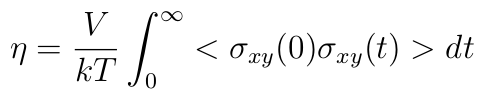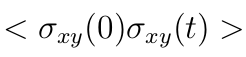# problems about ave/correlate and outputs

Dear all,

By citing the example, the following codes can be used to compute the viscosity from Green-Kubo methods ():

reset_timestep 0
variable pxy equal pxy
variable pxz equal pxz
variable pyz equal pyz
fix SS all ave/correlate \$s \$p d & v_pxy v_pxz v_pyz type auto file S0St.dat ave running variable scale equal {convert}/(\${kB}\$T)\$V* s*{dt}
variable v11 equal trap(f_SS){scale} variable v22 equal trap(f_SS)*{scale}
variable v33 equal trap(f_SS)
\${scale}
thermo_style custom step temp press v_pxy v_pxz v_pyz v_v11 v_v22 v_v33
run 600000
variable v equal (v_v11+v_v22+v_v33)/3.0

And right now, I just want to generate the result of, no integral is needed, how should I revised the codes? I changes the codes as below, but error occurred. How should I change the codes? I read the lammps mannual several times, however, I still can not get the point.
variable

reset_timestep 0
variable pxy equal pxy
variable pxz equal pxz
variable pyz equal pyz
fix SS all ave/correlate \$s \$p d & v_pxy v_pxz v_pyz type auto file S0St.dat ave running variable scale equal {convert}/(\${kB}\$T)V variable v11 equal f_SS*{scale}
variable v22 equal f_SS*{scale} variable v33 equal f_SS*{scale}

Your help will be greatly appreciated!!!

Dear all,

By citing the example, the following codes can be used to compute the
viscosity from Green-Kubo methods ():

...
reset_timestep 0
variable pxy equal pxy
variable pxz equal pxz
variable pyz equal pyz
fix SS all ave/correlate \$s \$p d &amp; &nbsp;&nbsp;&nbsp;&nbsp;&nbsp;&nbsp;&nbsp;&nbsp;&nbsp;&nbsp;&nbsp;&nbsp;&nbsp;v\_pxy v\_pxz v\_pyz type auto file S0St\.dat ave running variable scale equal {convert}/(\${kB}*\$T)*\$V* s\*{dt}
variable v11 equal trap(f_SS)*\{scale\} variable v22 equal trap\(f\_SS\[4\]\)\*{scale}
variable v33 equal trap(f_SS)*\${scale}
thermo_style custom step temp press v_pxy v_pxz v_pyz v_v11 v_v22 v_v33
run 600000
variable v equal (v_v11+v_v22+v_v33)/3.0

And right now, I just want to generate the result of , no integral is
needed, how should I revised the codes? I changes the codes as below, but
error occurred. How should I change the codes? I read the lammps mannual
several times, however, I still can not get the point.

​what error?​

variable

​the term your describe is not a point but a list, as it is a function of
time t, which is then discretized ​with using discrete time steps.

​axel.​